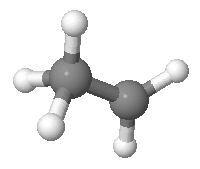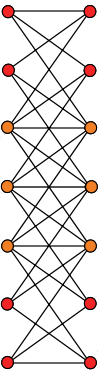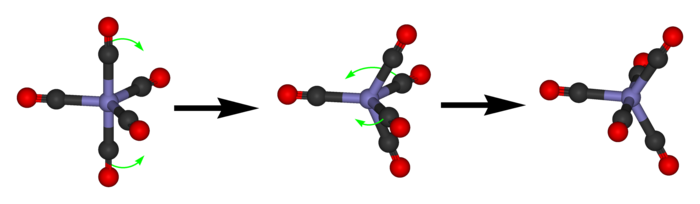# The Azimuth Project Blog - network theory (part 15)

This page is a blog article in progress, written by John Baez. To see discussions of it as it was being written, go to the Azimuth Forum. To see the final polished version, visit the Azimuth Blog.

Last time we saw how to get a graph whose vertices are states of a molecule and whose edges are transitions between states. We focused on two beautiful but not completely realistic examples that both give rise to the same highly symmetrical graph: the ‘Desargues graph’.

Today I’ll start with a few remarks about the Desargues graph. Then I’ll get to work showing how any graph gives:

• A Markov process, namely a random walk on the graph.
• A quantum process, where instead of having a probability to hop from vertex to vertex as time passes, we have an amplitude.

The trick is to use an operator called the ‘graph Laplacian’, a discretized version of the Laplacian which happens to be both infinitesimal stochastic and self-adjoint. As we saw in Part 12, such an operator will give rise both to a Markov process and a quantum process, by which I mean a one-parameter unitary group.

The most famous operator that’s both infinitesimal stochastic and self-adjoint is the Laplacian, $\nabla^2$. Because it’s both, the Laplacian shows up in two important equations: one in stochastic mechanics, the other in quantum mechanics.

$\frac{d}{d t} \psi = \nabla^2 \psi$

describes how the probability $\psi(x)$ of a particle being at the point $x$ smears out as the particle randomly walks around:The corresponding Markov process is called ‘Brownian motion’.$\frac{d}{d t} \psi = -i \nabla^2 \psi$

describes how the amplitude $\psi(x)$ of a particle being at the point $x$ wiggles about as the particle ‘quantumly’ walks around.

Both these equations have analogues where we replace space by a graph, and today I’ll describe them.

#### Drawing the Desargues graph

First I want to show you a nice way to draw the Desargues graph. For this it’s probably easiest to go back to our naive model of an ethyl cation:Even though ethyl cations don’t really look like this, and we should be talking about some trigonal bipyramidal molecule instead, it won’t affect the math to come. Mathematically, the two problems are isomorphic! So let’s stick with this nice simple picture.

We can be a bit more abstract, though. A state of the ethyl cation is like having 5 balls, with 3 in one pile and 2 in the other. And we can focus on the first pile and forget the second, because whatever isn’t in the first pile must be in the second.

Of course a mathematician calls a pile of things a ‘set’, and calls those things ‘elements’. So let’s say we’ve got a set with 5 elements. Draw a red dot for each 2-element subset, and a blue dot for each 3-element subset. Draw an edge between a red dot and a blue dot whenever the 2-element subset is contained in the 3-element subset. We get the Desargues graph.

That’s true by definition. But I never proved that the pictures I showed you are correct! For example, this picture shows the Desargues graph:

but I didn’t really prove it—and I won’t now, either.

To draw a picture we know is correct, it’s actually easier to start with a big graph that has vertices for all the subsets of our 5-element set. If we draw an edge whenever an $n$-element subset is contained in an $(n+1)$-element subset, the Desargues graph will be sitting inside this big graph.

Here’s what the big graph looks like:This graph has $2^5$ vertices. It’s actually a picture of a 5-dimensional cube! The vertices are arranged in columns. There’s

• one 0-element subset,

• five 1-element subsets,

• ten 2-element subsets,

• ten 3-element subsets,

• five 4-element subsets,

• one 5-element subset.

So, the numbers of vertices in each column go like this:

$1 \quad 5 \quad 10 \quad 10 \quad 5 \quad 1$

which is a row in Pascal’s triangle. We get the Desargues graph if we keep only the vertices corresponding to 2- and 3-element subsets, like this:It’s less pretty than our earlier picture, but at least there’s no mystery to it. Also, it shows that the Desargues graph can be generalized in various ways. For example, there’s a theory of bipartite Kneser graphs $H(n,k)$. The Desargues graph is $H(5,2)$.

#### Desargues' theorem

This is an even bigger digression. Why is it called the ‘Desargues graph’? This name comes from Desargues’ theorem, a famous result in projective geometry. Suppose you have two triangles ABC and abc, like this:

Suppose the lines Aa, Bb, and Cc all meet at a single point, the ‘center of perspectivity’. Then the point of intersection of ab and AB, the point of intersection of ac and AC, and the point of intersection of bc and BC all lie on a single line, the ‘axis of perspectivity’! The converse is true too. Quite amazing!

The Desargues configuration consists of all the actors in this drama:

• 10 points: A, B, C, a, b, c, the center of perspectivity, and the three points on the axis of perspectivity

and

• 10 lines: Aa, Bb, Cc, AB, AC, BC, ab, ac, bc and the axis of perspectivity

Given any configuration of points and lines, we can form a graph called its Levi graph by drawing a vertex for each point or line, and drawing an edge whenever a point lies on a line. And now for the punchline: Levi graph of the Desargues configuration is the ‘Desargues-Levi graph’!—or Desargues graph, for short.

Alas, I don’t know how this is relevant to anything I’ve discussed. For now it’s just a tantalizing curiosity.

#### A random walk on the Desargues graph

Back to business! I’ve been telling you about the analogy between quantum mechanics and stochastic mechanics. This analogy becomes especially interesting in chemistry, which lies on the uneasy borderline between quantum and stochastic mechanics.

Fundamentally, of course, atoms and molecules are described by quantum mechanics. But sometimes chemists describe chemical reactions using stochastic mechanics instead. When can they get away with this? Apparently whenever the molecules involved are big enough and interacting with their environment enough for ‘decoherence’ to kick in. I won’t attempt to explain this now.

Let’s imagine we have a molecule of iron pentacarbonyl with—here’s the unrealistic part, but it’s not really too bad—distinguishable carbonyl groups:

Iron pentacarbonyl is liquid at room temperatures, so as time passes, each molecule will bounce around and occasionally do a maneuver called a ‘pseudorotation’:We can approximately describe this process as a random walk on a graph whose vertices are states of our molecule, and whose edges correspond to transitions between states—namely, pseudorotations. And as we saw last time , this graph is the Desargues graph:Note, all the transitions are reversible here. And thanks to the enormous amount of symmetry, the rates of all these transitions must be equal.

Let’s write $V$ for the set of vertices of the Desargues graph. A probability distribution of states of our molecule is a function

$\psi : V \to [0,\infty)$

with

$\sum_{x \in V} \psi(x) = 1$

We can think of these probability distributions as living in this vector space:

$L^1(V) = \{ \psi: V \to \mathbb{R} \}$

I’m calling this space $L^1$ because of the general abstract nonsense explained in Part 12: probability distributions on any measure space live in a vector space called $L^1$. Today that notation is overkill, since every function on $V$ lies in $L^1$. But please humor me.

The point is that we’ve got a general setup that applies here. There’s a Hamiltonian:

$H : L^1(V) \to L^1(V)$

describing the rate at which the molecule randomly hops from one state to another… and the probability distribution $\psi \in L^1(X)$ evolves in time according to the equation

$\frac{d}{d t} \psi(t) = H \psi(t)$

But what’s the Hamiltonian $H$? It’s very simple, because it’s equally likely for the state to hop from any vertex to any other vertex that’s connected to that one by an edge. Why? Because the problem has so much symmetry that nothing else makes sense.

So, let’s write $E$ for the set of edges of the Desargues graph. We can think of this as a subset of $V \times V$, by saying $(x,y) \in E$ when $x$ is connected to $y$ by an edge. Then

$(H \psi)(x) = \sum_{y: (x,y) \in E} \psi(y) \quad - \quad 3 \psi(x)$

We’re subtracting $3 \psi(x)$ because there are 3 edges coming out of each vertex $x$, so this is the rate at which the probability of staying at $x$ decreases. We could multiply this Hamiltonian by a constant if we wanted the random walk to happen faster or slower… but let’s not.

The next step is to solve the equation

$\frac{d}{d t} \psi(t) = H \psi(t)$

Abstractly, the solution is easy:

$\psi(t) = \exp(t H) \psi(0)$

But to actually compute $\exp(t H)$, we might want to diagonalize the operator $H$. In this particular example, we could take advantage of the enormous symmetry of the Desargues graph. Its symmetry group includes the permutation group $S_5$, so we could take the vector space $L^1(V)$ and break it up into irreducible representations of $S_5$. Each of these will give an eigenspace of $H$, so by this method we can diagonalize $H$. I’d sort of like to try this… but it’s a big digression, so I won’t. At least, not today!

#### Graph Laplacians

The Hamiltonian we just saw is an example of a graph Laplacian. We can write down such a Hamiltonian for any graph, but it gets a tiny bit more complicated when different vertices have different numbers of edges coming out of them.

The word ‘graph’ means lots of things, but right now I’m talking about simple graphs. Such a graph has a set of vertices $V$ and a set of edges $E \subseteq V \times V$, such that

$(x,y) \in E \implies (y,x) \in E$

which says the edges are undirected, and

$(x,x) \notin E$

which says there are no loops. Let $d(x)$ be the degree of the vertex $x$, meaning the number of edges coming out of it.

Then the graph Laplacian is this operator on $L^1(V)$:

$(H \psi)(x) = \sum_{y: (x,y) \in E} \Psi(y) \quad - \quad d(x) \Psi(x)$

There is a huge amount to say about graph Laplacians! If you want, you can get started here:

But for now, let’s just say that $\exp(t H)$ is a Markov process describing a random walk on the graph, where hopping from one vertex to any neighboring vertex has unit probability per unit time. We can make the hopping faster or slower by multiplying $H$ by a constant. And here is a good time to admit that most people use a graph Laplacian that’s the negative of ours, and write time evolution as $\exp(-t H)$. The advantage is that then the eigenvalues of the Laplacian are $\ge 0$.

But what matters most is this. We can write the operator $H$ as a matrix whose entry $H_{x y}$ is 1 when there’s an edge from $x$ to $y$ and 0 otherwise—except when $x = y$, in which case the entry is $-d(x)$.

And then:

Puzzle 1. Show that for any finite graph, the graph Laplacian $H$ is infinitesimal stochastic, meaning that:

$\sum_{x \in V} H_{x y} = 0$

and

$x \ne y \implies H_{x y} \ge 0$

This fact implies that for any $t \ge 0$, the operator $\exp(t H)$ is stochastic—just what we need for a Markov process.

But we could also use $H$ as a Hamiltonian for a quantum system, if we wanted. Now we let $\psi(x)$ be the amplitude for being in the state $x \in V$. But now $\psi$ is a function

$\psi : V \to \mathbb{C}$

with

$\sum_{x \in V} |\psi(x)|^2 = 1$

We can think of this function as living in the Hilbert space

$L^2(V) = \{ \psi: V \to \mathbb{C} \}$

where the inner product is

$\langle \phi, \psi \rangle = \sum_{x \in V} \overline{\phi(x)} \psi(x)$

Puzzle 2. Show that for any finite graph, the graph Laplacian $H: L^2(V) \to L^2(V)$ is self-adjoint, meaning that:

$H_{x y} = \overline{H}_{y x}$

This implies that for any $t \in \mathbb{R}$, the operator $\exp(-i t H)$ is unitary—just what we need for one-parameter unitary group. So, we can take this version of Schrödinger’s equation:

$\frac{d}{d t} \psi = -i H \psi$

and solve it:

$\psi(t) = \exp(-i t H) \psi(0)$

and we’ll know that time evolution is unitary.

category: blog